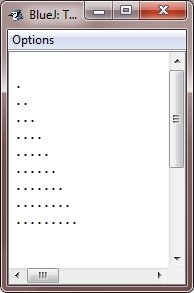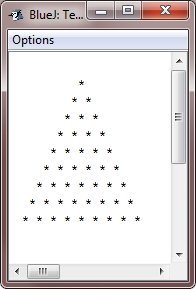# Java Nested Loops

Java allows loops to be nested (i.e., loops within loops) like all the other programming languages allows.

Nested loops means loops within loops. In other words, nested loops means, loop inside loop inside loop and so on.

## Java Nested Loops Example

Following is an example program that nests the Java for loops:

```/* Java Program Example - Java Nested Loops */

public class JavaProgram
{
public static void main(String args[])
{

int i, j;

for(i=0; i<10; i++)
{
for(j=0; j<i; j++)
{
System.out.print(".");
}
System.out.println();
}

}
}```

When the above Java program is compile and executed, it will produce the following output:Let's look at one more example which also demonstrate the nested loops:

```/* Java Program Example - Java nested loops */

public class JavaProgram
{
public static void main(String args[])
{

int i, j, k, space=10;

for(i=0; i<10; i++)
{
for(k=0; k<space; k++)
{
System.out.print(" ");
}

for(j=0; j<i; j++)
{
System.out.print("* ");
}
System.out.println();

space--;
}

}
}```

The sample run of the above Java program is shown here:### More Examples

Here are the list of some example programs that used nested loops:

Tools
Calculator